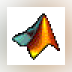### MATLAB R2012a

MATLAB is a programming environment for algorithm development, data analysis, visualization, and numerical computation.### MATLAB R2016a

MATLAB (Matrix Laboratory) is a mathematical and scientific computational tool.### MATLAB Student

MATLAB is the language of technical computing at leading engineering and science companies and the standard software at more than 5000 universities worldwide.### MATLAB R2015a (32-bit)

MATLAB (Matrix Laboratory) is a simulation package for engineering applications.### MATLAB R2015a

MATLAB (Matrix Laboratory) is a simulation package for engineering applications.### MatLab CAPE-OPEN Thermo Import

This Matlab package allows for importing CAPE-OPEN version 1.

... calculations inside Matlab. The package ... -bits, or version 7.1 or higher, 64-bits ...### Motion Lab Systems C3Dserver

5 on 1 vote

C3Dserver is a 32-bit C3D Software Development Kit (SDK) for Microsoft Windows environments.

... is a 32-bit C3D Software ... with 32-bit MATLAB and Lab ... 32-bit app's in a 64-bit environment ...### Frontline Systems Solver Platform SDK

Solver Platform SDK is a powerful, comprehensive Software Development Kit that enables you to develop and deploy custom ...

... , Java and MATLAB, as well ... 64-bit and 32-bit Windows and 64-bit ...### MATLAB Compiler Runtime

The MATLAB Runtime is a standalone set of shared libraries that enables the execution of compiled MATLAB applications ...

... MATLAB installed. When used together, MATLAB, MATLAB ...### MATLAB Component Runtime

MATLAB Component Runtime is a standalone set of shared libraries that enable the execution of M-files.

... for the MATLAB language, ... for MATLAB Compiler ... NET objects. MATLAB Component Runtime ...### MATLAB Production Server

MATLAB Production Server lets you run MATLAB programs within your production systems, enabling you to incorporate custom analytics in enterprise applications.

... run MATLAB programs ... applications request MATLAB analytics ... running on MATLAB Production ...### NeuroSolutions for MATLAB

NeuroSolutions for MATLAB neural network toolbox is a MATLAB add-in that is easy-to-use and intuitive.

... MATLAB neural network toolbox is a MATLAB ... intelligence inside MATLAB. The software ...The Matlab Structs Tool (aka 'loaddap') provides a way to read any DAP-accessible data into Matlab.

The Matlab Structs Tool ( ... data into Matlab. This is ... by creating Matlab Structure variables ...### Ideal MP3 Music Sorter

Ideal MP3 Music Sorter - will sort, organize and rename all your Music files, fast and easy.

... ), SoundFoundry WAVE 64 (w64),Matlab (mat ...